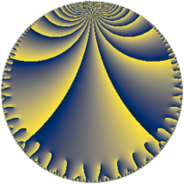# Properties

 Label 91.8.bcLevel $91$ Weight $8$ Character orbit 91.bc Rep. character $\chi_{91}(6,\cdot)$ Character field $\Q(\zeta_{12})$ Dimension $256$ Newform subspaces $1$ Sturm bound $74$ Trace bound $0$

# Related objects

## Defining parameters

 Level: $$N$$ $$=$$ $$91 = 7 \cdot 13$$ Weight: $$k$$ $$=$$ $$8$$ Character orbit: $$[\chi]$$ $$=$$ 91.bc (of order $$12$$ and degree $$4$$) Character conductor: $$\operatorname{cond}(\chi)$$ $$=$$ $$91$$ Character field: $$\Q(\zeta_{12})$$ Newform subspaces: $$1$$ Sturm bound: $$74$$ Trace bound: $$0$$

## Dimensions

The following table gives the dimensions of various subspaces of $$M_{8}(91, [\chi])$$.

Total New Old
Modular forms 272 272 0
Cusp forms 256 256 0
Eisenstein series 16 16 0

## Trace form

 $$256 q - 8 q^{2} - 12 q^{4} - 2576 q^{7} - 520 q^{8} + 90392 q^{9} + O(q^{10})$$ $$256 q - 8 q^{2} - 12 q^{4} - 2576 q^{7} - 520 q^{8} + 90392 q^{9} + 140 q^{11} + 18952 q^{14} - 5144 q^{15} + 524284 q^{16} - 49780 q^{18} + 105576 q^{21} - 16068 q^{22} - 12 q^{23} - 82212 q^{28} - 515084 q^{29} - 807756 q^{30} + 664108 q^{32} - 828764 q^{35} - 907920 q^{36} + 336116 q^{37} + 1230908 q^{39} - 2861572 q^{42} + 1445760 q^{43} + 6188328 q^{44} + 7251012 q^{46} - 150672 q^{49} + 6746164 q^{50} - 13243992 q^{53} + 12393168 q^{56} - 6634220 q^{57} - 12046944 q^{58} + 10702124 q^{60} - 13227040 q^{63} + 2971492 q^{65} + 17955336 q^{67} + 14991480 q^{70} + 29025828 q^{71} - 24700280 q^{72} + 2492856 q^{74} - 49919332 q^{78} + 15493808 q^{79} - 47979668 q^{81} - 57449120 q^{84} + 44075300 q^{85} + 52959360 q^{86} + 52919340 q^{88} + 21040608 q^{91} - 132973464 q^{92} + 113964348 q^{93} - 30386388 q^{95} - 71663372 q^{98} + 7655092 q^{99} + O(q^{100})$$

## Decomposition of $$S_{8}^{\mathrm{new}}(91, [\chi])$$ into newform subspaces

Label Dim $A$ Field CM Traces $q$-expansion
$a_{2}$ $a_{3}$ $a_{5}$ $a_{7}$
91.8.bc.a $256$ $28.427$ None $$-8$$ $$0$$ $$0$$ $$-2576$$